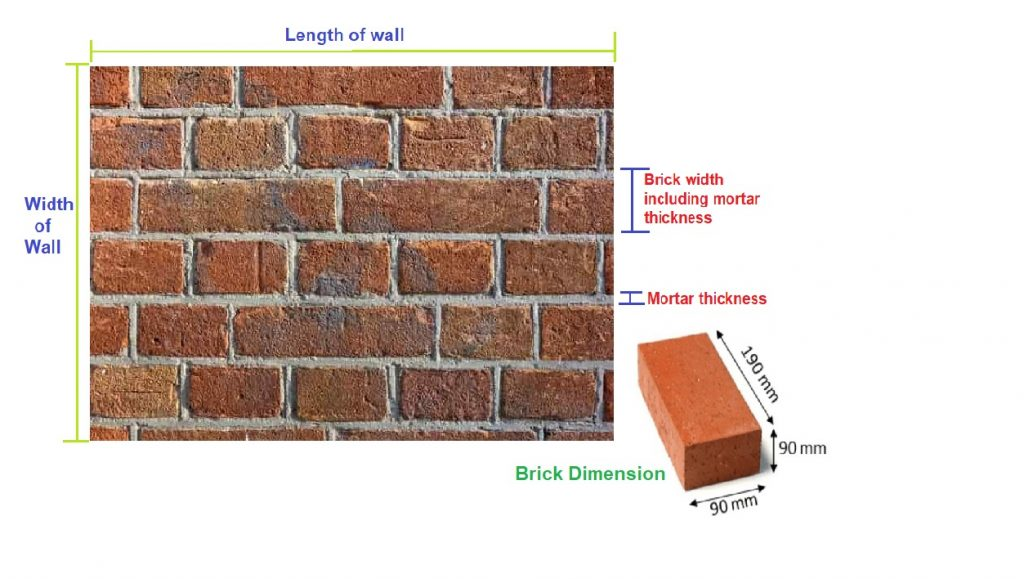Work Hours
Monday to Friday: 7AM - 7PM
Weekend: 10AM - 5PM

# Brick Calculator For Wall

## Calculations for Brick Calculator for Wall

Let’s understand with the help of an example, how to use the above Calculations for Brick Calculator for WallAssumptions For Example

• Brick Dimension = 0.19 x 0.09 x 0.09 m
• Length of wall = 10 m
• Width of wall = 10 m
• Thickness of wall = 0.23 m
• Cement:Sand Ratio = 1:6
• Mortar Thickness = 0.01 m
• Wastage of mortar = 10%
• Volume of 1 cement bag = 0.035 m3

Calculation Procedure

• Step 1: Calculation of Volume
Volume of wall = 10 x 10 x 0.23
= 23 m3
Volume of brick = 0.19 x 0.09 x 0.09
= 0.001539 m3
Volume of brick with mortar thickness = (0.19+0.01) x (0.09+0.01) x (0.09+0.01)
= 0.2 x 0.1 x 0.1 = 0.002 m3

• Step 2: Calculation of the number of bricks
No. of brick = Volume of wall / Volume of brick including mortar thickness
= 23 / 0.002
= 11500 bricks

• Step 3: Calculation of Mortar volume(wet & dry)
Mortar volume required = (Volume of wall – (Volume of brick without mortar thickness x No. of brick))
= 23 – (0.001539 x 11500)
= 5.30 m3
Adding 10% wastage,Required mortar = 5.30 + 10% of 5.30= 5.83 m3
Here above mortar is wet volume of mortar
Dry Volume of Mortar = Wet volume x 1.33
= 5.83 x 1.33
= 7.75 m3

• Step 4: Calculation of Cement and Sand
Since Cement: Sand = 1:6
The volume of cement = (1 x volume of dry mortar) / 7
= (1x 7.75) / 7
= 1.10 m3
No. of Cement Bags = Volume of cement / Volume of 1 bag of cement
= 1.10 / 0.035
= 32 Bags
The volume of sand = (6 x volume of dry mortar) / 7
= (6x 7.75) / 7
= 6.65 m3

Final Result

Number of Bricks = 11500 Bricks
Number of Cement Bags = 32 Bags
Volume of Sand = 6.65 m3

Try Onsite, #1 Tool For Site Tracking

/** zohoform script */